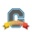Community Participant

## "weigh all assignments in a group the same"

I need the ability to "weigh all assignments in a group the same" so that assignments with different point values all have the same weight.

I cannot design all my assignments to have the same point value. This would mean they all use the same or similar rubric, and they do not.

The only way to do this is to enter percentage points instead of actual points... then they all have a "100" value. This is more time consuming.

Labels (1)
• ### weighted assignments in groups

2 RepliesCommunity Coach

the following is not a solution, but a possible work around.  The only issue (other than the extra work) is the confusion to the students.

For the assignment group, add a 0 point assignment called grade correction (you could put your explanation in the assignment description with an example calculation).  furthermore, this idea works best after all assignments are finished and not necessarily something you would want to do after each assignment is turned in - although it could be done.

Export the gradebook and change the csv file to an xlsx file and remove all assignment and grade information except for the group in question.  Then you have to come up with formula to calculate the grade correction value for the assignments present.  At this point, without more information from you, I can only guess what you are trying to do with all assignments weighted the same.  I'll use an example of 4 assignments so each assignment is worth 25% of the grade for the group. So the percent grade for the group will be 100%

#1 - 18/20 = 90% ==> group points 25*.9 = 22.5 (25% of 90 )
#2 - 12/15 = 80% ==> group points of 25*.8 = 20
#3 - 10/10 = 100% ==> group points of 25*1.0 = 25
#4 - 15/25 = 60% ==> group points of 25*.6 = 15

Therefore total points for the group should be 82.5/100 or 82.5%,  For the actual assignments, the student score is 55/70 = 78.57%  so to get to 82.5%  the correction point score is (.825 - 0.7857)*70 = 2.75.  Adding in 2.75 points to the student score gives them 57.75/70 = 82.5%.  then you just have to import the correction score

Once you have it setup in a spread sheet in excel (I would use a cell for holding the number of assignments and use it for determining the weight for each assignment), set up the equations to calculate the points .  Then as each assignment is completed you should be able to insert a new column for that assignment, put in those grades and update the assignment count to get the correction points which are then imported over the existing ones.

Not an ideal case, but once it is setup and you have done it once or twice, the time to do it will go fairly quick.  Unfortunately, another issue will be that as students drop the course, you will have to update the student name list each time as well.

good luck

RonCommunity Champion

I know you stated that you couldn't set all your assignments to be worth the same points, but I think that's likely the easiest solution.  Set all the assignments to be worth the same amount of points by adjusting the different point values of the criterions on the rubric. If, in the end, they are weighted the same, then individual criterion values aren't as important overall, they are only important as they relate to other criterion on the rubric. That is, on each rubric you are indicating to students what work is more valuable than others.  But, overall each assignment is worth the same.

For example, if all assignments are worth 25 points:

Rubric 1 with 3 criteria,

1=10 pts

2=8 pts

3=7 pts

Rubric 2 with 4 criteria,

1=5 pts

2=5 pts

3=7.5 pts

4=7.5 pts

It's pretty easy to change the rubrics by adjusting each criterion's value, the tool auto adjust each rating value.

Just a thought.  It would be some work to edit them, but once done, you'd have it.